# Formulas of arithmetical and geometrical progressions

## Arithmetic progression:

### Progression's elements:

a1, a2, a3, ... , an

d

### Progression's element calculation formulas:

ai = ai+1d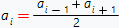an = a1 + (n1) d

### Sum of the first n-elements of progression formulas: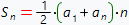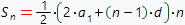## Geometric progression:

### Progression's elements:

b1, b2, b3, ... , bn

### Progression's denominator:

q, if | q | < 1, then progression is called infinitely decreasing progression

### Progression's element calculation formulas:

bi+1 = bi q
bi2 = b i1 b i + 1

bn = b1 q (n1)

### Sum of the first n-elements of progression formula: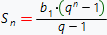### Sum of the infinitely decreasing progression's formula: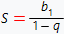, | q | < 1

## Other useful links:

Step by step solution of partial derivative sample
Logarithms formulas
Step by step solution of parametric derivative